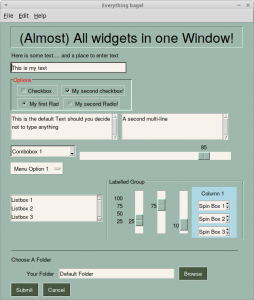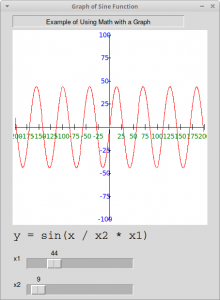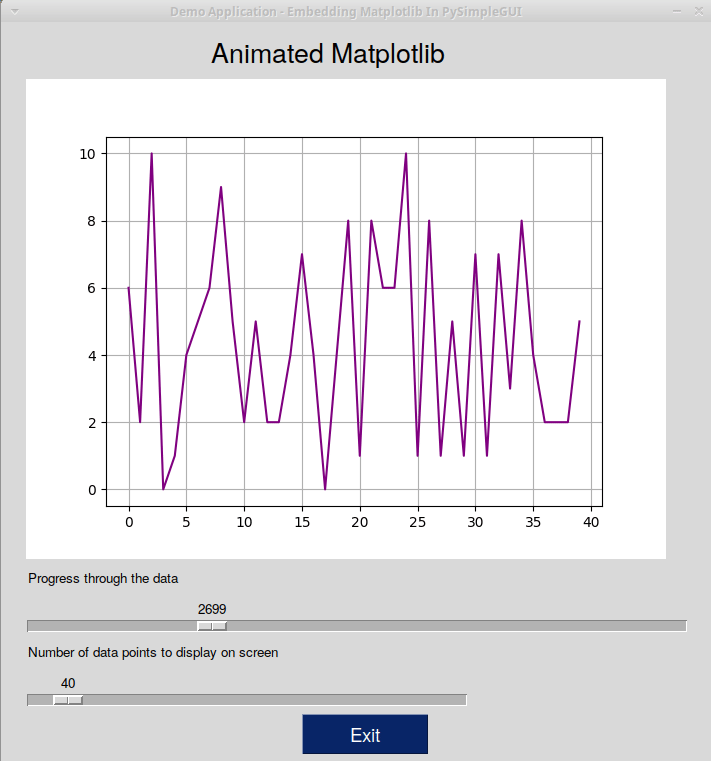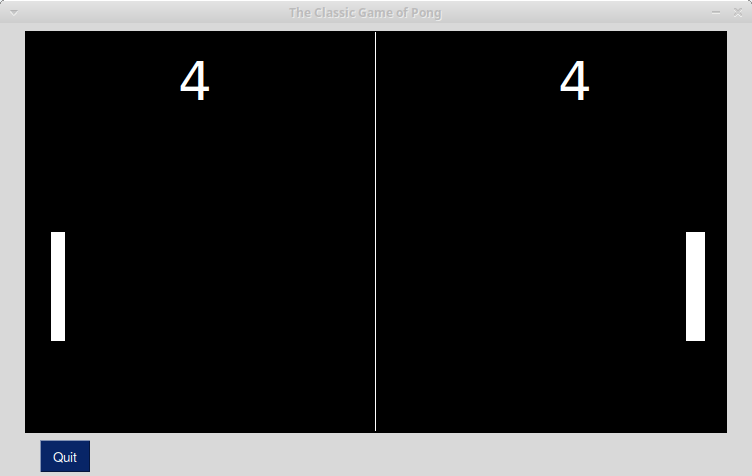# The Demos for PySimpleGUI

The PySimpleGUI project has a lot of interesting demos included with their project that you can use to learn how to use PySimpleGUI. The demos cover all the basic widgets as far as I can tell and they also cover the recommended design patterns for the package. In addition, there are a couple of games and other tiny applications too, such as a version of Pong and the Snake game.

In this article, you will see a small sampling of the demos from the project that will give you some idea of what you can do with PySimpleGUI.

### Seeing the Available Widgets

PySimpleGUI has a nice little demo called Demo_All_Widgets.py that demonstrates almost all the widgets that PySimpleGUI supports currently. PySimpleGUI has wrapped all of Tkinter’s core widgets, but not the ttk widgets.

This is what the demo looks like when you run it:Let’s take a quick look at the code for this demo:

```#!/usr/bin/env python
'''
Example of (almost) all widgets, that you can use in PySimpleGUI.
'''

import PySimpleGUI as sg

sg.change_look_and_feel('GreenTan')

# ------ Menu Definition ------ #
menu_def = [['&File', ['&Open', '&Save', 'E&xit', 'Properties']],
['&Edit', ['Paste', ['Special', 'Normal', ], 'Undo'], ],

# ------ Column Definition ------ #
column1 = [[sg.Text('Column 1', background_color='lightblue', justification='center', size=(10, 1))],
[sg.Spin(values=('Spin Box 1', '2', '3'),
initial_value='Spin Box 1')],
[sg.Spin(values=('Spin Box 1', '2', '3'),
initial_value='Spin Box 2')],
[sg.Spin(values=('Spin Box 1', '2', '3'), initial_value='Spin Box 3')]]

layout = [
[sg.Text('(Almost) All widgets in one Window!', size=(
30, 1), justification='center', font=("Helvetica", 25), relief=sg.RELIEF_RIDGE)],
[sg.Text('Here is some text.... and a place to enter text')],
[sg.InputText('This is my text')],
[sg.Frame(layout=[
[sg.CBox('Checkbox', size=(10, 1)),
sg.CBox('My second checkbox!', default=True)],
title_color='red',
relief=sg.RELIEF_SUNKEN,
tooltip='Use these to set flags')],
[sg.MLine(default_text='This is the default Text should you decide not to type anything', size=(35, 3)),
sg.MLine(default_text='A second multi-line', size=(35, 3))],
[sg.Combo(('Combobox 1', 'Combobox 2'), size=(20, 1)),
sg.Slider(range=(1, 100), orientation='h', size=(34, 20), default_value=85)],
[sg.Listbox(values=('Listbox 1', 'Listbox 2', 'Listbox 3'), size=(30, 3)),
sg.Frame('Labelled Group', [[
sg.Slider(range=(1, 100), orientation='v', size=(5, 20), default_value=25, tick_interval=25),
sg.Slider(range=(1, 100), orientation='v', size=(5, 20), default_value=75),
sg.Slider(range=(1, 100), orientation='v', size=(5, 20), default_value=10),
sg.Col(column1, background_color='lightblue')]])
],
[sg.Text('_' * 80)],
[sg.Text('Choose A Folder', size=(35, 1))],
sg.InputText('Default Folder'), sg.FolderBrowse()],
[sg.Submit(tooltip='Click to submit this form'), sg.Cancel()]]

window = sg.Window('Everything bagel', layout,
default_element_size=(40, 1), grab_anywhere=False)

sg.popup('Title',
'The results of the window.',
'The button clicked was "{}"'.format(event),
'The values are', values)
```

PySimpleGUI lays out their widgets by using Python lists. You can also see that this demo uses lists for generating the menus too. Then you create a Window object and pass in the layout, which is your list of lists of Elements or widgets.

Let’s see what else you can do!

### Graphing with PySimpleGUI

PySimpleGUI also supports creating graphs. One such example can be found in Demo_Graph_Element_Sine_Wave.py. This demo shows the developer how to use the Graph widget.

This is what the demo looks like when you run it:Here is what the code looks like:

```import PySimpleGUI as sg
import math

# Yet another usage of Graph element.

SIZE_X = 200
SIZE_Y = 100
NUMBER_MARKER_FREQUENCY = 25

def draw_axis():
graph.draw_line((-SIZE_X, 0), (SIZE_X, 0))                # axis lines
graph.draw_line((0, -SIZE_Y), (0, SIZE_Y))

for x in range(-SIZE_X, SIZE_X+1, NUMBER_MARKER_FREQUENCY):
graph.draw_line((x, -3), (x, 3))                       # tick marks
if x != 0:
# numeric labels
graph.draw_text(str(x), (x, -10), color='green')

for y in range(-SIZE_Y, SIZE_Y+1, NUMBER_MARKER_FREQUENCY):
graph.draw_line((-3, y), (3, y))
if y != 0:
graph.draw_text(str(y), (-10, y), color='blue')

# Create the graph that will be put into the window
graph = sg.Graph(canvas_size=(400, 400),
graph_bottom_left=(-(SIZE_X+5), -(SIZE_Y+5)),
graph_top_right=(SIZE_X+5, SIZE_Y+5),
background_color='white',
key='graph')
# Window layout
layout = [[sg.Text('Example of Using Math with a Graph', justification='center', size=(50, 1), relief=sg.RELIEF_SUNKEN)],
[graph],
[sg.Text('y = sin(x / x2 * x1)', font='COURIER 18')],
[sg.Text('x1'), sg.Slider((0, 200), orientation='h',
enable_events=True, key='-SLIDER-')],
[sg.Text('x2'), sg.Slider((1, 200), orientation='h', enable_events=True, key='-SLIDER2-')]]

window = sg.Window('Graph of Sine Function', layout)

while True:
if event is None:
break
graph.erase()
draw_axis()
prev_x = prev_y = None

for x in range(-SIZE_X, SIZE_X):
y = math.sin(x/int(values['-SLIDER2-']))*int(values['-SLIDER-'])
if prev_x is not None:
graph.draw_line((prev_x, prev_y), (x, y), color='red')
prev_x, prev_y = x, y

window.close()
```

To make the graph work correctly, you need to erase the graph and redraw it in the while loop above. Play around with the code a bit and see what you can do. There are several other graph related demos in the demo folder that you should check out as well.

PySimpleGUI also supports matplotlib integration. A fun one to play around with is Demo_Matplotlib_Animated.py.

When I ran it, the demo ended up looking like this:Now let’s check out another demo!

### Creating Pong with PySimpleGUI

As I mentioned earlier in this article, you can also create the Pong game pretty easily using PySimpleGUI. You can check out Demo_Pong.py for full details.

Here is what the code creates when you run it:The code for this game is a bit long, but not too hard to follow. At the time of writing, the game is written using 183 lines of code in a single module.

### Wrapping Up

There are 150+ demos in PySimpleGUI’s Demo folder. I did discover a few that didn’t work on Linux due to using OS-specific code. However most of the examples seem to work and they are a great way to see what you can do with this project. Check them out to get some ideas of how you could use PySimpleGUI for your own projects or demos.